Next: SSA in buffers Up: Reducing Spurious Switching Activity Previous: Overhead for gating

## Different output network topologies

Three output network topologies were introduced in Section III. If all the output data are sent to all the receivers without regard to whether they need the data or not, the MST output network will consume the least power and the fully dedicated the most. If interconnect SSA is suppressed using the proposed techniques, however, this will not always be true. Fig. 10 shows a DPU, colored black, sending data to two other DPUs, colored white, using different output network topologies. It also shows the optimal locations for gating signals with SSA as small triangles.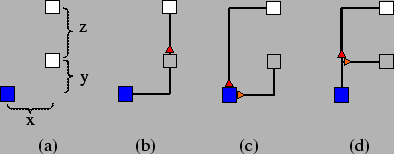Suppose tri-state buffers are used so that the last useful value is remembered in the gated idle cycles. Let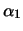denote the unit-length switched capacitance for data, say, for variables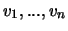, needed by the upper receiver, which is estimated as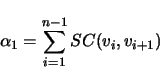where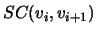is the unit-length switched capacitance in the wire when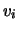is followed by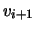. That for the lower receiver,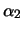, and that for all the output variables of the sender,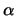, can be estimated in the same way. Assuming signal gating is performed at the optimal locations shown in Fig. 10, we can estimate the total wire switched capacitance for the output network implemented as follows: MST,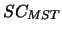, fully dedicated,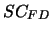, and trunk-branches,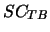, as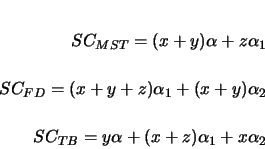The above equations imply that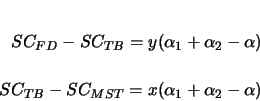Therefore, when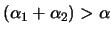, we haveand when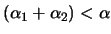,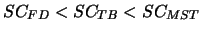. Since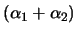can be either larger or smaller than, none of these topologies is always better than the others in terms of power consumption. In this work, the trunk-branches topology is assumed due to its never-the-worst power consumption and the algorithmic simplicity for constructing it.Next: SSA in buffers Up: Reducing Spurious Switching Activity Previous: Overhead for gating
Lin Zhong 2003-10-11